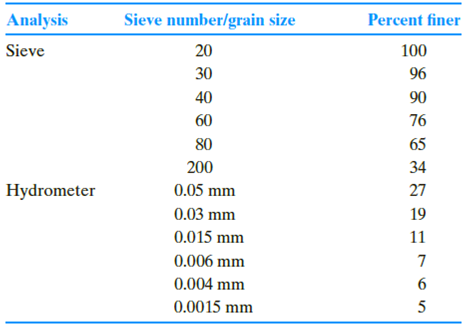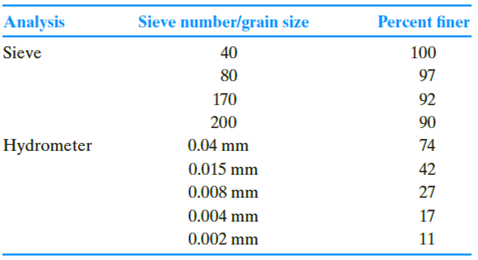Chapter 2, Problem 2.10PPrinciples of Geotechnical Enginee...

9th Edition
Braja M. Das + 1 other
ISBN: 9781305970939

Solutions

Chapter
SectionPrinciples of Geotechnical Enginee...

9th Edition
Braja M. Das + 1 other
ISBN: 9781305970939
Textbook Problem

Repeat Problem 2.8 using the following data.2.8 The following are the results of a sieve and hydrometer analysis.a. Draw the grain-size distribution curve.b. Determine the percentages of gravel, sand, silt and clay according to the MIT system.c. Repeat Part b according to the USDA system.d. Repeat Part b according to the AASHTO system.

(a)

To determine

Draw the grain-size distribution curve.

Explanation

Given Information:

The sieve and hydrometer analysis are given in the Table.

The percent finer value for corresponding grain size is given in Table.

Calculation:

Plot the grain size distribution curve using grain size versus percent finer...

(b)

To determine

Calculate the percentages of gravel, sand, silt, and clay according to the MIT system.

(c)

To determine

Calculate the percentages of gravel, sand, silt, and clay according to the USDA system.

(d)

To determine

Calculate the percentages of gravel, sand, silt, and clay according to the AASTHO system.

Still sussing out bartleby?

Check out a sample textbook solution.

See a sample solution

The Solution to Your Study Problems

Bartleby provides explanations to thousands of textbook problems written by our experts, many with advanced degrees!

Get Started

Give examples of the role of motors in our daily life.

Engineering Fundamentals: An Introduction to Engineering (MindTap Course List)

What is residual risk?

Principles of Information Security (MindTap Course List)

What does the term 3 Vs refer to?

Database Systems: Design, Implementation, & Management

Describe how to perform a starter bench test.

Automotive Technology: A Systems Approach (MindTap Course List)

Determine the resultant of the four forces.

International Edition---engineering Mechanics: Statics, 4th Edition

Same as Problem 8.6-2, but use ASD.

Steel Design (Activate Learning with these NEW titles from Engineering!)